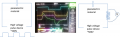# Can I trust this high voltage supply instrument ?

#### hwoarang

Joined Dec 28, 2015
18
Hello, everyone.
Now I am doing some experiments where the high voltage pulse (500V with 2.5 kHz) is applied on the capacitor (piezoelectric material, exactly) like the drawing below (left one). I want to know how fast the piezoelectric materials can be charged. Actually, this high voltage supply instrument supports voltage and current monitoring channels which can be connected to oscilloscope. The middle picture means the voltage profile (pink) and current profile (green) which were measured using these monitoring channels. But I cannot trust these profiles because even after the voltage is saturated, the current has a long tail. If this is a simple RC circuit, the current would be similar to the derivative of the voltage response (I(t) ~ dV(t)/dt). So, I add the resistor in the middle of this circuit (right figure) to measure the voltage for the test. The yellow graph in the middle picture is this voltage profile. What I don't understand is that why the yellow and pink graphs are same. In principle, after the capacitor is fully charged, no current flows in the circuit. This means that the yellow graph should decay to zero like current response (green graph) during capacitor's charging, I think. Or, does the current flow constantly even after full charge in the capacitor ? What is wrong here..? Can I trust the current reponse (green graph) at least ?